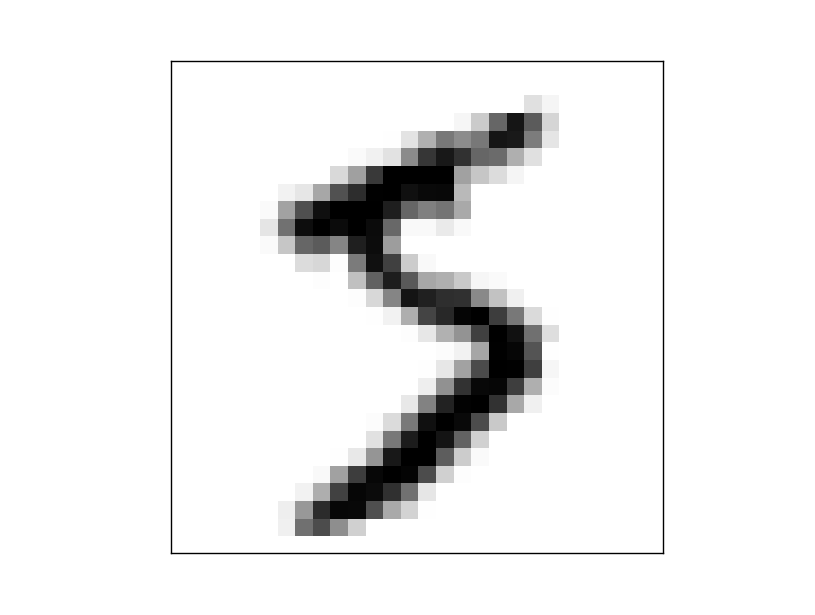After using CNN in previous article, it still can’t recognize the correct name of birds if the little creature stand on the corner (instead of the center) of the whole picture. Then I started to think about the problem: how to let neural-network ignore the position of the bird in picture, but only focus on its exists? Eventually I recollected the “max pooling”:From: http://mxnet.io/tutorials/python/mnist.html

By choose the max feature value from 2×2 pad, it will amplify the most important feature without affected by backgrounds. For example, if we split a picture into 2×2 chassis (4 plates) and the bird only stand in the first plate, the “max pooling” will choose only the first plate for next processing. Those trees, pools, leaves and other trivial issues in other three plates will be omitted.
Then I modify the structure of CNN again:

```def convolution_network():
data = mx.sym.Variable('data')
conv1 = mx.sym.Convolution(data=data, kernel=(12, 12), stride=(5, 5), num_filter=128)
bn1 = mx.sym.BatchNorm(data=conv1, fix_gamma=False, eps=2e-5, momentum=0.9, name="bn1")
tanh1 = mx.sym.Activation(data=bn1, act_type="relu")
pool1 = mx.sym.Pooling(data=tanh1, pool_type="max", kernel=(2,2), stride=(2,2))
conv2 = mx.sym.Convolution(data=pool1, kernel=(12, 12), stride=(5, 5), num_filter=128)
bn2 = mx.sym.BatchNorm(data=conv2, fix_gamma=False, eps=2e-5, momentum=0.9, name="bn2")
tanh2 = mx.sym.Activation(data=bn2, act_type="relu")
pool2 = mx.sym.Pooling(data=tanh2, pool_type="max", kernel=(2,2), stride=(2,2))
fc3 = mx.sym.FullyConnected(data=pool2, num_hidden=3)
return mx.sym.SoftmaxOutput(data=fc3, name='softmax')
```

and using “0.3” for my learning rate, as “0.3” is better to against overfitting.
For one week (Chinese New Year Festival), I was studying “Neural Networks and Deep Learning”. This book is briefly awesome! A lot of doubts about Neural Networks for me have been explained and resolved. In third chapter, the author Michael Nielsen suggests a method, which really enlightened me, to defeat overfitting: artificially expanding training data. The example is rotating the MNIST handwritten digital picture by 15 degrees:In my case, I decided to crop different parts of bird picture if the picture is a rectangle:by using the python PIL (Picture Processing Library):

```def crop_image(origin, imgs, box):
result = origin.crop(box)
result.thumbnail((edge, edge), Image.NEAREST)
imgs.append(result)
def crop_and_append_image(img, imgs):
tp = img.getbbox()
width = tp
height = tp
if (width > height):
sub = width - height
crop_image(img, imgs, (sub / 2, 0, height + sub / 2, height))
if (sub >= 80):
crop_image(img, imgs, (sub / 2 - 40, 0, height + sub / 2 - 40, height))
crop_image(img, imgs, (sub / 2 + 40, 0, height + sub / 2 + 40, height))
elif (height > width):
sub = height - width
crop_image(img, imgs, (0, sub / 2, width, width + sub / 2))
if (sub >= 80):
crop_image(img, imgs, (0, sub / 2 - 40, width, width + sub / 2 - 40))
crop_image(img, imgs, (0, sub / 2 + 40, width, width + sub / 2 + 40))
else:
img.thumbnail((edge, edge), Image.NEAREST)
imgs.append(img)
```

The effect of using “max pooling” and “expanding training data” is significant: